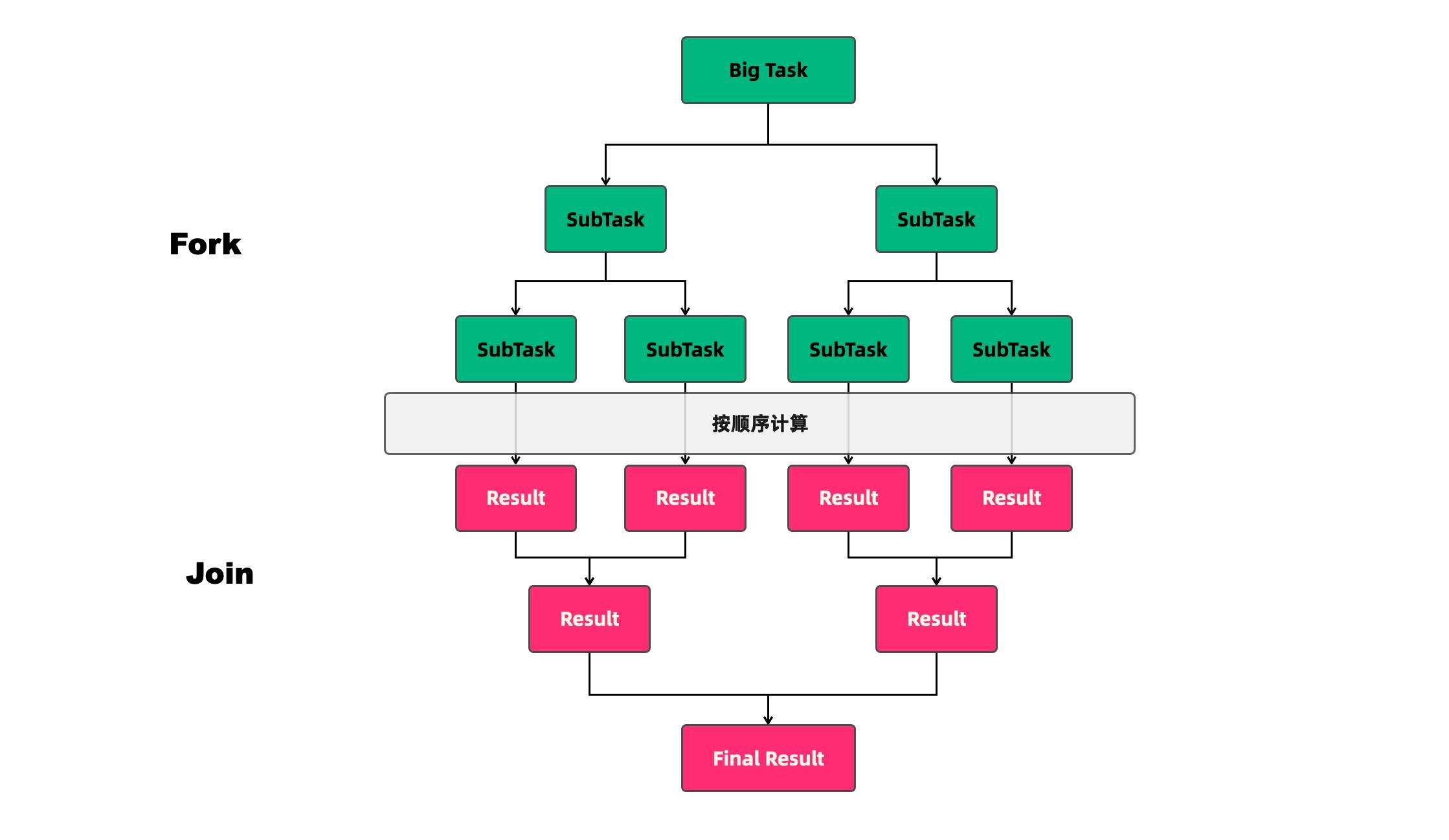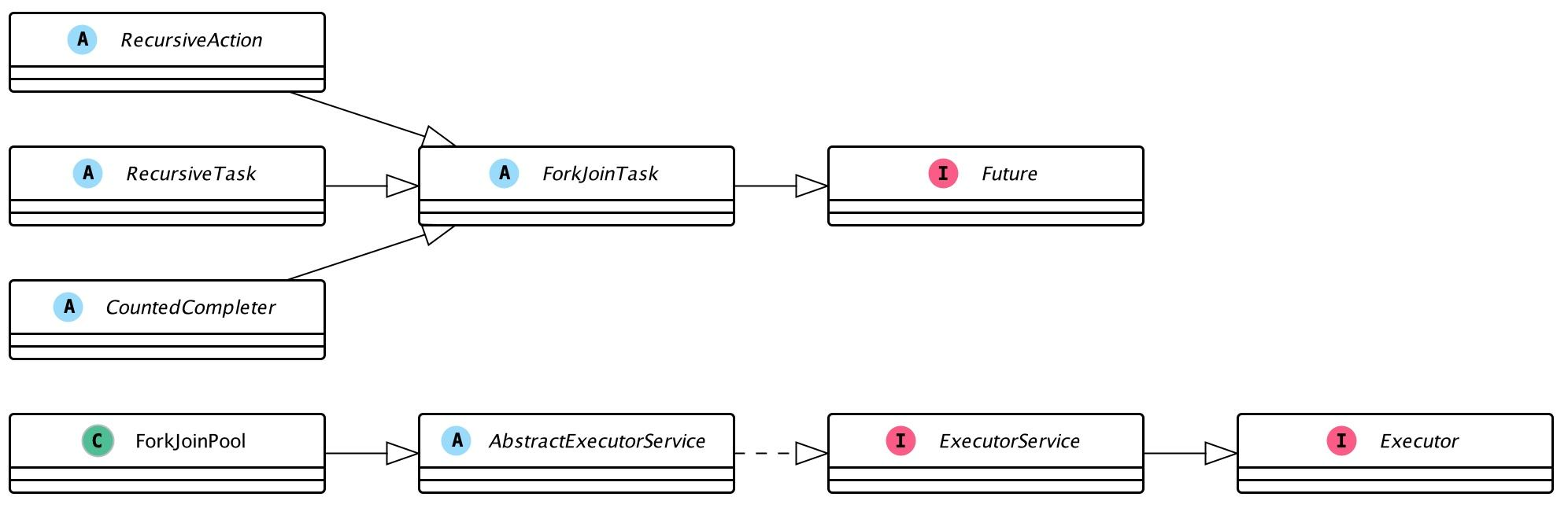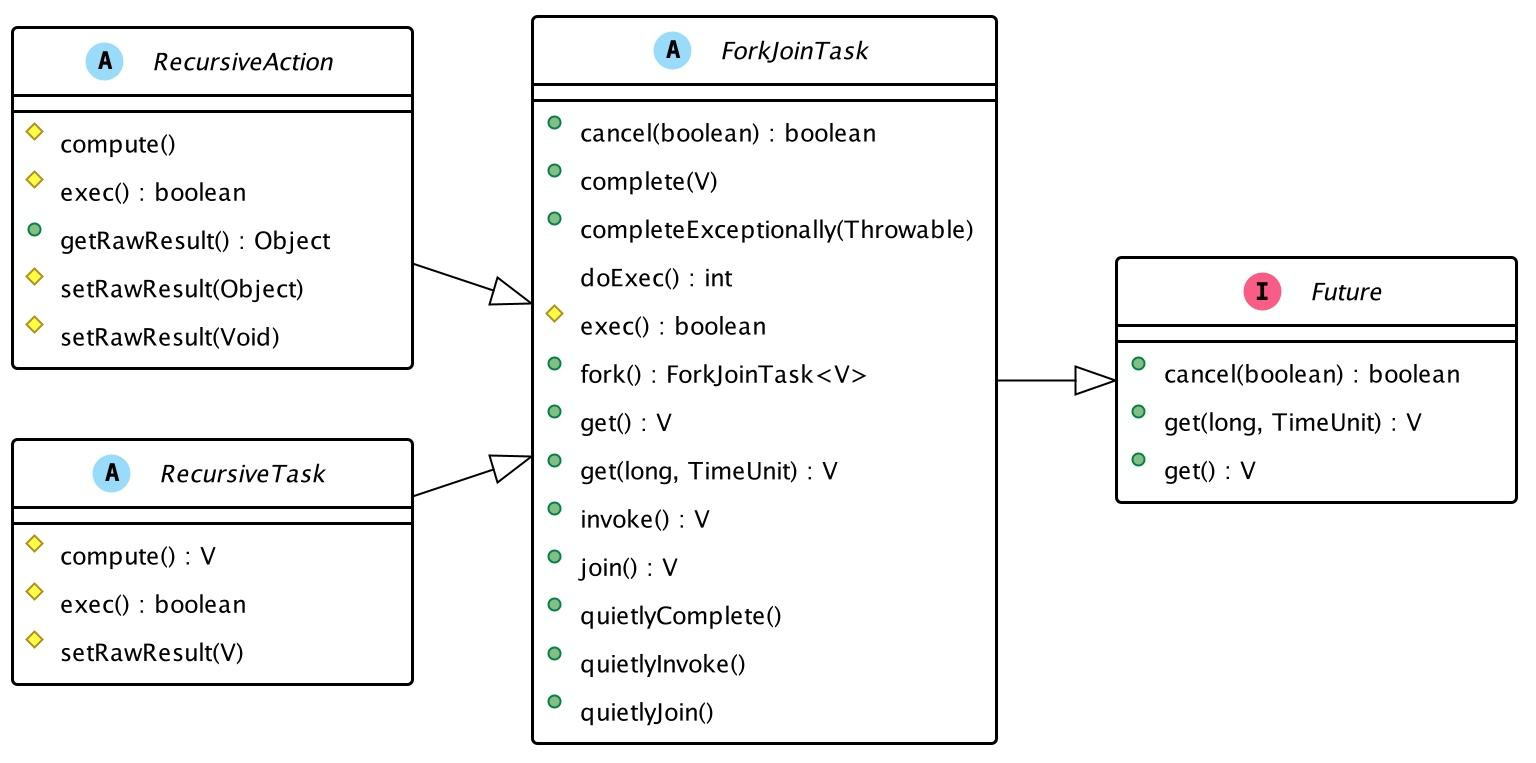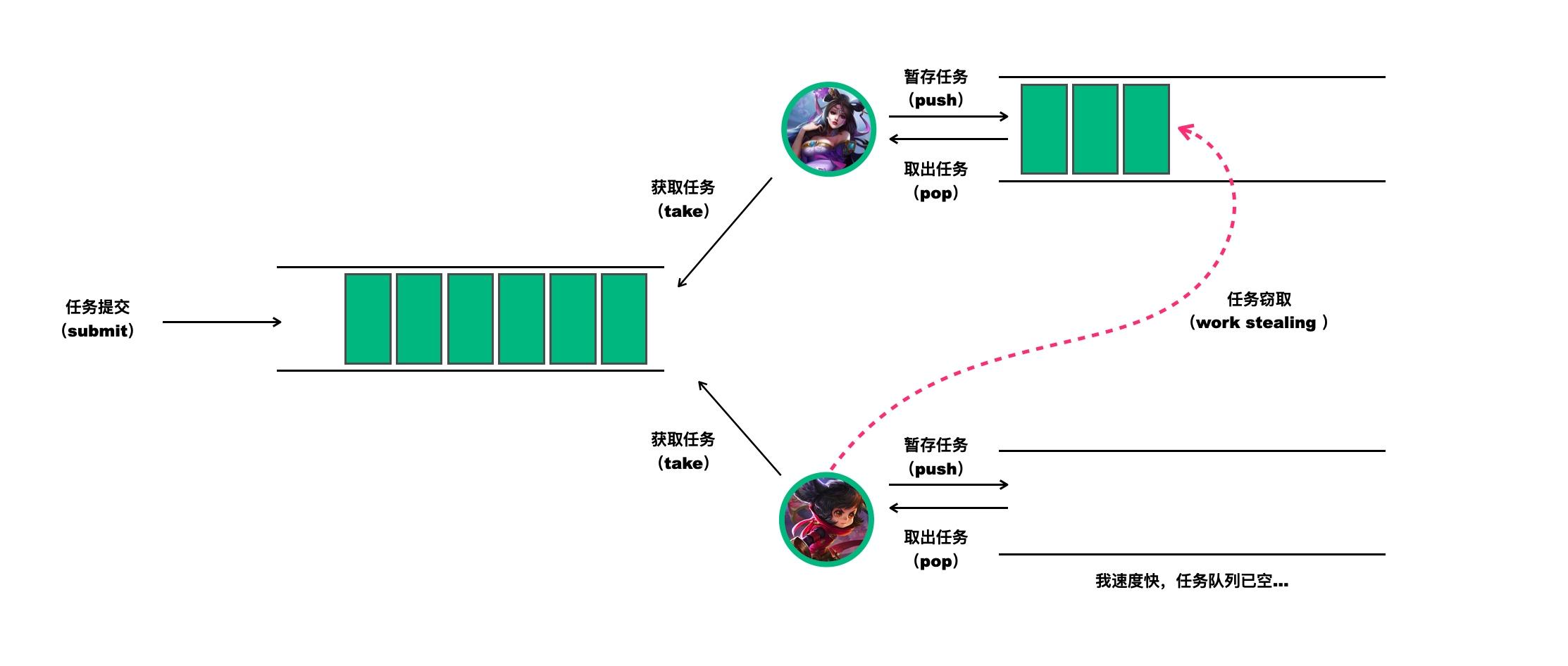# 王者并发课-钻石2：分而治之-如何从原理深入理解ForkJoinPool的快与慢

2022-06-13 19:54 2141 查看

## 一、分治算法与Fork/Join模式

• （1）分解：将要解决的问题划分成若干规模较小的同类问题；
• （2）求解：当子问题划分得足够小时，用较简单的方法解决；
• （3）合并：按原问题的要求，将子问题的解逐层合并构成原问题的解。Fork/Join对任务的拆分和对结果合并过程也是如此，可以用下面伪代码来表示：

```solve(problem):
if problem is small enough:
// 如果任务足够小，执行任务
solve problem directly (sequential algorithm)
else:
// 拆分任务
for part in subdivide(problem)
// 合并结果
join all subtasks spawned in previous loop
return combined results```

## 二、Fork/Join应用场景与体验

`sumBegin`
`sumEnd`
）和拆分阈值（
`threshold`
），以及核心计算逻辑
`compute()`
.

```public class TheKingRecursiveSumTask extends RecursiveTask<Long> {
private static final AtomicInteger taskCount = new AtomicInteger();
private final int sumBegin;
private final int sumEnd;
/**
* 任务拆分阈值，当任务尺寸大于该值时，进行拆分
*/
private final int threshold;

public TheKingRecursiveSumTask(int sumBegin, int sumEnd, int threshold) {
this.sumBegin = sumBegin;
this.sumEnd = sumEnd;
this.threshold = threshold;
}

@Override
protected Long compute() {
if ((sumEnd - sumBegin) > threshold) {
// 两个数之间的差值大于阈值，拆分任务
}
// 直接执行结果
long result = 0L;
for (int i = sumBegin; i < sumEnd; i++) {
result += i;
}
return result;
}

}
}```

```public static void main(String[] args) {
int sumBegin = 0, sumEnd = 10000000;
computeByForkJoin(sumBegin, sumEnd);
}

private static void computeByForkJoin(int sumBegin, int sumEnd) {
ForkJoinPool forkJoinPool = new ForkJoinPool(16);
long forkJoinStartTime = System.nanoTime();
System.out.println("======");
System.out.println("ForkJoin计算结果：" + forkJoinResult);
System.out.println("ForkJoin计算耗时：" + (System.nanoTime() - forkJoinStartTime) / 1000000);
}

private static void computeBySingleThread(int sumBegin, int sumEnd) {
long computeResult = 0 L;
long startTime = System.nanoTime();
for (int i = sumBegin; i < sumEnd; i++) {
computeResult += i;
}
System.out.println("======");
System.out.println("单线程计算结果：" + computeResult);
System.out.println("单线程计算耗时：" + (System.nanoTime() - startTime) / 1000000);
}```

```======
ForkJoin任务拆分：131071
ForkJoin计算结果：49999995000000
ForkJoin计算耗时：207
======

Process finished with exit code 0```

## 三、ForkJoinPool设计与源码分析### 1. 构造ForkJoinPool的几种不同方式

ForkJoinPool中有四个核心参数，用于控制线程池的并行数工作线程的创建异常处理模式指定等。各参数解释如下：

• `int parallelism`
：指定并行级别（parallelism level）。ForkJoinPool将根据这个设定，决定工作线程的数量。如果未设置的话，将使用
`Runtime.getRuntime().availableProcessors()`
来设置并行级别；
• `ForkJoinWorkerThreadFactory factory`
• `UncaughtExceptionHandler handler`
：指定异常处理器，当任务在运行中出错时，将由设定的处理器处理；
• `boolean asyncMode`
：从名字上看，你可能会觉得它是异步模式设置，但其实是设置队列的工作模式：
`asyncMode ? FIFO_QUEUE : LIFO_QUEUE`
. 当asyncMode为true时，将使用先进先出队列，而为false时则使用后进先出的模式。

（1）方式一：默认无参构造

```public ForkJoinPool() {
this(Math.min(MAX_CAP, Runtime.getRuntime().availableProcessors()),
}```

（2）方式二：通过并行数构造

```public ForkJoinPool(int parallelism) {
}```

（2）方式三：自定义全部参数构造

```public ForkJoinPool(int parallelism,
UncaughtExceptionHandler handler,
boolean asyncMode) {
this(checkParallelism(parallelism),
checkFactory(factory),
handler,
asyncMode ? FIFO_QUEUE : LIFO_QUEUE,
"ForkJoinPool-" + nextPoolId() + "-worker-");
checkPermission();
}```

### 2. 按类型提交不同任务

（1）第一类核心方法：invoke

```public <T> T invoke(ForkJoinTask<T> task) {
throw new NullPointerException();
}```

（2）第二类核心方法：execute

```public void execute(ForkJoinTask<?> task) {
throw new NullPointerException();
}

throw new NullPointerException();
else
externalPush(job);
}```

（3）第三类核心方法：submit

```public < T > ForkJoinTask < T > submit(ForkJoinTask < T > task) {
throw new NullPointerException();
}

public < T > ForkJoinTask < T > submit(Callable < T > task) {
externalPush(job);
return job;
}

public < T > ForkJoinTask < T > submit(Runnable task, T result) {
externalPush(job);
return job;
}

throw new NullPointerException();
else
externalPush(job);
return job;
}```#### （1）fork与join

`fork()`
/
`join()`

fork-提交任务

`fork()`

`subTask1.fork()`
. 注意，不同于其他线程池的写法，任务提交由任务自己通过调用
`fork()`

`fork()`

```public final ForkJoinTask<V> fork() {
else
ForkJoinPool.common.externalPush(this);
return this;
}```

join-获取任务执行结果

`fork()`

`join()`

`join()`

`join()`

`doJoin()`

`doJoin()`

```public final V join() {
int s;
// 如果调用doJoin返回的非NORMAL状态，将报告异常
if ((s = doJoin() & DONE_MASK) != NORMAL)
reportException(s);
// 正常执行结束，返回原始结果
return getRawResult();
}

private int doJoin() {
int s;
ForkJoinPool.WorkQueue w;
//如果已完成，返回状态
return (s = status) < 0 ? s :
(w = (wt = (ForkJoinWorkerThread) t).workQueue).
tryUnpush(this) && (s = doExec()) < 0 ? s :
wt.pool.awaitJoin(w, this, 0 L):
externalAwaitDone();
}

final int doExec() {
int s;
boolean completed;
if ((s = status) >= 0) {
try {
completed = exec();
} catch (Throwable rex) {
return setExceptionalCompletion(rex);
}
// 执行完成后，将状态设置为NORMAL
if (completed)
s = setCompletion(NORMAL);
}
return s;
}```RecursiveAction：无结果返回

RecursiveAction用于递归执行但不需要返回结果的任务，比如下面的排序就是它的典型应用场景。在使用RecursiveAction时，你需要继承并实现它的核心方法

`compute()`
.

```static class SortTask extends RecursiveAction {
final long[] array;
final int lo, hi;
SortTask(long[] array, int lo, int hi) {
this.array = array;
this.lo = lo;
this.hi = hi;
}
this(array, 0, array.length);
}
// 核心计算方法
protected void compute() {
if (hi - lo < THRESHOLD)
// 直接执行
sortSequentially(lo, hi);
else {
// 拆分任务
int mid = (lo + hi) >>> 1;
merge(lo, mid, hi);
}
}
// implementation details follow:
static final int THRESHOLD = 1000;
void sortSequentially(int lo, int hi) {
Arrays.sort(array, lo, hi);
}
void merge(int lo, int mid, int hi) {
long[] buf = Arrays.copyOfRange(array, lo, mid);
for (int i = 0, j = lo, k = mid; i < buf.length; j++)
array[j] = (k == hi || buf[i] < array[k]) ?
buf[i++] : array[k++];
}
}```

`compute()`
.

```class Fibonacci extends RecursiveTask<Integer> {
final int n;
Fibonacci(int n) { this.n = n; }
Integer compute() {
if (n <= 1)
return n;
Fibonacci f1 = new Fibonacci(n - 1);
f1.fork();
Fibonacci f2 = new Fibonacci(n - 2);
return f2.compute() + f1.join();
}
}```

### 4. 工作队列与任务窃取

ForkJoinPool的大部分操作都发生在工作窃取队列（work-stealing queues ） 中，该队列由内部类WorkQueue实现。其实，这个队列也不是什么神奇之物，它是Deques的特殊形式，但仅支持三种操作方式：pushpoppoll（也称为窃取）。当然，在ForkJoinPool中，队列的读取有着严格的约束，

`push`
`pop`

`poll`### 5. ForkJoinPool监控

（1）获取运行状态的线程总数

```public int getRunningThreadCount() {
int rc = 0;
WorkQueue[] ws;
WorkQueue w;
if ((ws = workQueues) != null) {
for (int i = 1; i < ws.length; i += 2) {
if ((w = ws[i]) != null && w.isApparentlyUnblocked())
++rc;
}
}
return rc;
}```

（2）获取活跃线程数量

```public int getActiveThreadCount() {
int r = (config & SMASK) + (int)(ctl >> AC_SHIFT);
return (r <= 0) ? 0 : r; // suppress momentarily negative values
}```

（3）判断ForkJoinPool是否空闲

```public boolean isQuiescent() {
return (config & SMASK) + (int)(ctl >> AC_SHIFT) <= 0;
}```

（4）获取任务窃取数量

```public long getStealCount() {
AtomicLong sc = stealCounter;
long count = (sc == null) ? 0 L : sc.get();
WorkQueue[] ws;
WorkQueue w;
if ((ws = workQueues) != null) {
for (int i = 1; i < ws.length; i += 2) {
if ((w = ws[i]) != null)
count += w.nsteals;
}
}
return count;
}```

（5）获取队列中的任务数量

```public long getQueuedTaskCount() {
long count = 0;
WorkQueue[] ws;
WorkQueue w;
if ((ws = workQueues) != null) {
for (int i = 1; i < ws.length; i += 2) {
if ((w = ws[i]) != null)
count += w.queueSize();
}
}
return count;
}```

（6）获取已提交的任务数量

```public int getQueuedSubmissionCount() {
int count = 0;
WorkQueue[] ws;
WorkQueue w;
if ((ws = workQueues) != null) {
for (int i = 0; i < ws.length; i += 2) {
if ((w = ws[i]) != null)
count += w.queueSize();
}
}
return count;
}```

## 四、警惕ForkJoinPool#commonPool

ForkJoinPool中的commonPool设计初衷是为了降低线程池的重复创建，让一些任务共用同一个线程池，毕竟创建线程池和创建线程都是昂贵的。然而，凡事都有两面性，commonPool在某些场景下确实可以达到线程池复用的目的，但是，如果你决定与别人分享自己空间，那么当你想使用它的时候，它可能不再完全属于你。也就是说，当你想用commonPool时，它可能已经其他任务填满了。

## 五、ForkJoinPool性能评估

• 实验代码：本文第一部分的示例代码；
• 实验环境：Mac；
• JDK版本：8；
• 任务分隔阈值：100

Fork/Join 单线程 Fork/Join 单线程 Fork/Join 单线程
1 4 0 34 5 1157 313
2 3 0 34 6 848 344
3 5 0 16 9 1069 325
4 4 0 35 8 955 307
5 5 0 30 22 922 385

```======
ForkJoin任务拆分：16383
ForkJoin计算结果：499999999500000000
ForkJoin计算耗时：143
======

## 小结

• 任务切分：将大的任务分割成更小粒度的小任务，让更多的线程参与执行；
• 任务窃取：通过任务窃取，充分地利用空闲线程，并减少竞争。

## 夫子的试炼

• 动手：使用ForkJoinPool实现List数组排序。

## 延伸阅读与参考资料

• 《王者并发课》大纲与更新进度总览：https://juejin.cn/post/6967277362455150628
• A Java Fork/Join Framework（Doug Lea）：http://gee.cs.oswego.edu/dl/papers/fj.pdf
• Introduction to the Fork/Join Framework：https://www.pluralsight.com/guides/introduction-to-the-fork-join-framework
• The Unfairly Unknown ForkJoinPool：https://medium.com/swlh/the-unfairly-unknown-forkjoinpool-c262777def6a
• A Java? Fork-Join Calamity：http://coopsoft.com/ar/CalamityArticle.html